Courses

# RD Sharma Solutions - Chapter 13 - Proft, Loss, Discount and Value Added Tax (VAT) (Part -1), Class8 Class 8 Notes | EduRev

## RD Sharma Solutions for Class 8 Mathematics

Created by: Abhishek Kapoor

## Class 8 : RD Sharma Solutions - Chapter 13 - Proft, Loss, Discount and Value Added Tax (VAT) (Part -1), Class8 Class 8 Notes | EduRev

The document RD Sharma Solutions - Chapter 13 - Proft, Loss, Discount and Value Added Tax (VAT) (Part -1), Class8 Class 8 Notes | EduRev is a part of the Class 8 Course RD Sharma Solutions for Class 8 Mathematics.
All you need of Class 8 at this link: Class 8

PAGE NO 13.11:

Question 1:

A student buys a pen for Rs 90 and sells it for Rs 100. Find his gain and gain percent.

C.P of pen = Rs. 90

S.P of pen  =  Rs. 100

Gain = SP −CP

Gain = 100−90 = Rs. 10

Gain% =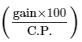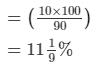PAGE NO 13.11:

Question 2:

Rekha bought a saree for Rs 1240 and sold it for Rs 1147. Find her loss and loss percent.

C.P of saree = Rs. 1240

S.P of saree  =  Rs. 1147

Loss = CP−SP

Loss =  Rs.(1240−1147) = Rs. 93

loss% =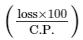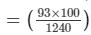= 7.5%

PAGE NO 13.11:

Question 3:

A boy buys 9 apples for Rs 9.60 and sells them at 11 for Rs 12. Find his gain or loss percent.

C.P of 9 apples = Rs 9.60

∴ C.P of 1 apple = Rs 9.609 = Rs16/15

S.P of 11 apples  =  Rs 12

∴ S.P of 1 apple = Rs 12/11

Clearly, S.P of 1 apple >C.P of 1 apple

So, we get profit on selling apples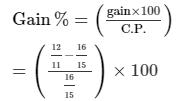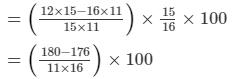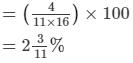PAGE NO 13.11:

Question 4:

The cost price of 10 articles is equal to the selling price of 9 articles. Find the profit percent.

Let the cost price of one article be Rs. C and the selling price be Rs. S

Therefore, 10C = 9S

C = 9/10.S

So, the cost price is less than the selling price.

S.P. =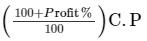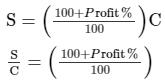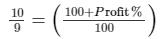1000/9 = 100 + Profit

00/9−100 = Profit%

Profit% =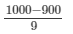=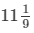Therefore, the required profit percent is

PAGE NO 13.11:

Question 5:

A retailer buys a radio for Rs 225. His overhead expenses are Rs 15. If he sells the radio for Rs 300, determine his profit percent.

C.P = Rs. (225 + 15) = Rs. 240

S.P = Rs. 300

Profit = SP−CP

=  Rs. (300−240)

= Rs. 60

Profit% = Profit/C.P × 100

= 60/240 × 100 = 25%

PAGE NO 13.11:

Question 6:

A retailer buys a cooler for Rs 1200 and overhead expenses on it are Rs 40. If he sells the cooler for Rs 1550, determine his profit percent.

Cooler costs =  Rs.1200

C.P = Rs. (1200 + 40) = Rs. 1240

S.P = Rs. 1550

Profit = SP−CP

= Rs. (1550−1240)

= Rs. 310

Profit% = Profit/C.P × 100

= 310/1240 × 100

= 25%

PAGE NO 13.11:

Question 7:

A dealer buys a wristwatch for Rs 225 and spends Rs 15 on its repairs. If he sells the same for Rs 300, find his profit percent.

A dealer buys a wrist watch for Rs. 225

Money spent on repairing the watch  = Rs. 15

Therefore,C.P = Rs. (225 + 15) = Rs. 240

S.P = Rs. 300

Profit = SP−CP

= Rs. (300−240)

= Rs. 60

Profit% = Profit/C.P × 100

= 60/240 × 100

= 25%

PAGE NO 13.11:

Question 8:

Ramesh bought two box es for Rs 1300. He sold one box at a profit of 20% and the other box at a loss of 12%. If the selling price of both box es is the same, find the cost price of each box .

Let the cost price of the first box  be Rs.  x .

Therefore, the cost of the second box  will be Rs. (1300− x )

Profit on the first box = 20 %

Loss on the second box = 12%

SP of the first box = CP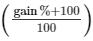SP = x (120/100)

SP of the  first box = Rs.120 x 100 = Rs.6 x 5

SP of the second box = CP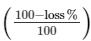S.P of the second box =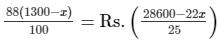Since S.P of both the box  are equal,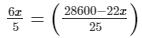150 x = 143000−110 x

260 x = 143000

x = 143000/260

x = 550

Therefore, the cost price of the first box  is Rs. 550.

The cost price of the second box  will be Rs.(1300−550) = Rs. 750

PAGE NO 13.11:

Question 9:

If the selling price of 10 pens is equal to cost price of 14 pens, find the gain percent.

Let the cost price of one pen be Rs. C, and the selling price be Rs. S

Therefore, 10S = 14C

C = 10/14.S

However, the cost price is less than the selling price.

S.P.  =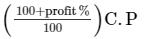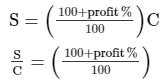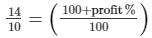1400/10 = 100 + profit%

140−100 = profit%

Profit% = 40 = 40%

Therefore, the required profit percent is 40%.

PAGE NO 13.11:

Question 10:

If the cost price of 18 chairs be equal to selling price of 16 chairs, find the gain or loss percent.

Let the cost price of one chair be Rs. C, and selling price be Rs. S

Therefore, 18C = 16S

C = 16/18.S

As cost price is less than the selling price,

S.P.  =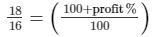1800/16 = 100 + profit%

180016−100 = profit%

Profit% =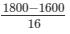= 200/16 = 12.5

Therefore, the required profit percent is 12.5%.

PAGE NO 13.11:

Question 11:

If the selling price of 18 oranges is equal to the cost price of 16 oranges, find the loss percent.

Let the cost price of one orange be Rs. C, and its selling price be Rs. S

Therefore, 16C = 18S

C = 18/16.S

As cost price is more than the selling price,

S.P.  =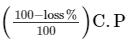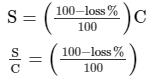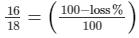1600/18 = 100−loss%

Loss% = 100−1600/18

Loss% =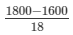= 200/18 = 100/9

=Therefore, the loss percent is%.

PAGE NO 13.11:

Question 12:

Ravish sold his motorcycle to Vineet at a loss of 28%. Vineet spent Rs 1680 on its repairs and sold the motor cycle to Rahul for Rs 35910, thereby making a profit of 12.5%, find the cost price of the motor cycle for Ravish.

Let the cost price of the motorcycle for Ravish be Rs.  x .

Loss% = 28%

Therefore, SP = CP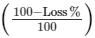SP = Rs.  x (72/100)

Selling price of the motorcycle for Ravish =  Cost  price of the motorcycle for Vineet

Money spent on repairs = Rs. 1680

Therefore, total cost price of the motorcycle for Vineet = Rs.(   x (72/100) + 1680)

Selling price of the motorcycle for Vineet  = Rs. 35910

Profit% = 12.5%

SP = CP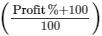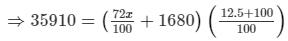⇒ 35910 × 100 × 100 = (72 x + 168000)(112.5)

⇒ 359100000 = 8100 x + 18900000

⇒ 340200000 = 8100 x

⇒ x = Rs. 42000

Therefore, Ravish bought the motorcycle for Rs. 42000

PAGE NO 13.12:

Question 13:

By selling a book for Rs 258, a bookseller gains 20%. For how much should he sell it to gain 30%?

Selling price of the book = Rs. 258

Gain% = 20%

Since, C.P =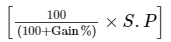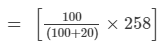= 25800/120

= Rs. 215

Let the bookseller sells it for Rs.  x

So, S.P  =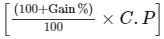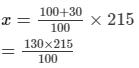= Rs. 279.50

Therefore, the bookseller must sell the book at Rs 279.50 to make 30% profit.

PAGE NO 13.12:

Question 14:

A defective briefcase costing Rs 800 is being sold at a loss of 8%. If the price is further reduced by 5%, find its selling price.

C.P of the briefcase = Rs. 800

Loss = 8%

S.P  = CP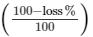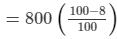= 800 × 0.92

= Rs. 736

If the price is further reduced by 5%, the selling price of the briefcase will be

=  Rs.(736−736 × 5/100)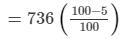= 736 × 0.95

= Rs. 699.20

Thus, the selling price of the briefcase will be Rs 699.20.

PAGE NO 13.12:

Question 15:

By selling 90 ball pens for Rs 160 a person loses 20%. How many ball pens should be sold for Rs 96 so as to have a profit of 20%?

S.P of 90 ball pens  =  Rs. 160

Loss = 20%

Therefore, C.P  = SP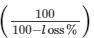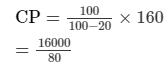= Rs. 200

Now,S.P of 90 ball pens = Rs. 96

Profit = 20%

C.P  = SP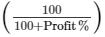CP =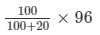= 9600/120

= Rs. 80

Rs. 200 is the C.P of 90 ball pens.

Therefore, Rs. 80 is the C.P of  =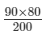= 36 ball pens

Thus, 36 ball pens should be sold at Rs. 96 to earn a profit of 20%.

PAGE NO 13.12:

Question 16:

A man sells an article at a profit of 25%. If he had bought it at 20% less and sold it for Rs 36.75 less, he would have gained 30%. Find the cost price of the article.

Let the C.P of the article be Rs.  x .

Original S.P  = x + 25/100.x

= Rs. 5x/4

If he purchased it at 20% less,C.P = x −20/100.x

= Rs. 4x/5

He sold the article at Rs 36.75 less.

So, the selling price  = Rs. 5x/4−36.75

Given that he would have gained 30% selling at that price.

Therefore, gain% =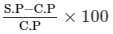S.P−C.P = 5x/4−36.75−4 x 5

= 5x/4−4x/5−36.75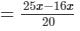−36.75

= 9x/20−36.75

So, gain% =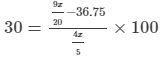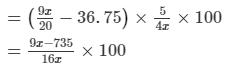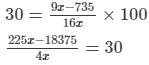225 x −18375 = 120 x

105 x = 18375 x

= 18375/105

= 175

So, the cost price of the article is Rs. 175.

PAGE NO 13.12:

Question 17:

A dishonest shopkeeper professes to sell pulses at his cost price but uses a false weight of 950 gm for each kilogram. Find his gain percent.

He sells 950gm pulses and gets a gain of 50gm.

If he sells 100gm of pulses, he will gain  = 509/50 × 100

= 5000/950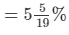PAGE NO 13.12:

Question 18:

A dealer bought two tables for Rs 3120. He sold one of them at a loss of 15% and other at a gain of 36%. Then, he found that each table was sold for the same price. Find the cost price of each table.

Given that the selling price is same for both the tables.

Let the C.P of 1 table be Rs.  x , then the C.P of the other will be Rs.(3120− x ).

Loss on the first table =  15%

Therefore, S.P = C.P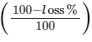S.P =  85x/100 = Rs. 0.85 x

Gain on the second table  =  36%

Therefore, S.P  = C.P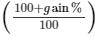S.P = Rs. 1.36(3120− x )

Since both tables have the same S.P,

So, 0.85 x = 1.36(3120− x )

0.85 x = 4243.20−1.36 x

2.21 x = 4243.20

x = 4243.20/2.21

x = Rs. 1920

So,the cost price of the first table is Rs. 1920.

Cost price of the second table  =  Rs. ( 3120−1920) = Rs. 1200

PAGE NO 13.12:

Question 19:

Mariam bought two fans for Rs 3605. She sold one at a profit of 15% and the other at a loss of 9%. If Mariam obtained the same amount for each fan, find the cost price of each fan.

It is given that the S.P is same for both the fans.Let C.P of the first fan be Rs.  x

Therefore, C.P of the second fan = Rs. (3605− x )

Profit on the first fan = 15%

Loss on the second fan = 9%

For the first fan,S.P  =  C.P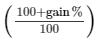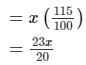For the second fan,S.P  =  C.P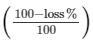= (3605− x )(91/100)

Since S.P of both the fans is the same,23x/20 = (3605− x )(91/100)

2300 x = 91(72/100−20 x )2300

x = 6561100−1820 x

4120 x = 6561100

x = Rs.1592.50

Thus, C.P of the first fan is Rs. 1592.50.C.P of the second fan  =  Rs. (3605−1592.50)             = Rs. 2012.50

PAGE NO 13.12:

Question 20:

Some toffees are bought at the rate of 11 for Rs 10 and the same number at the rate of 9 for Rs 10. If the whole lot is sold at one rupee per toffee, find the gain or loss percent on the whole transaction.

Let the total number of toffees bought be  x.

Let  x/2 toffees at the rate of 11 are bought for Rs.10, and  x/2 toffees at the rate of 9 are bought for Rs.10

Total money spent on buying the toffees =  (x/2)(10/11) + (x/2)(10/9)

= 200x/198

= 100/99 x

It is given that  x  toffees are sold at one rupee per toffee.

Therefore, the selling price of  x  toffees  = Rs.  x × 1 = Rs.  x

As C.P is more than S.P, it will be a loss.

Loss = C.P−S.P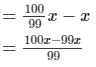= x/99

Loss% = Loss/C.P × 100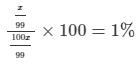Total loss on the whole transaction would be 1%.

PAGE NO 13.12:

Question 21:

A tricycle is sold at a gain of 16%. Had it been sold for Rs 100 more, the gain would have been 20%. Find the C.P. of the tricycle.

Let the S.P of the tricycle be Rs.  x  and C.P be Rs. y Gain% = 16%

S.P = C.P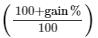Then we have, x = y +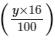x = y + 0.16y

x = 1.16y

When S.P increases by Rs. 100,

we get

⇒ x + 100 = y +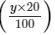Putting  x = 1.6y, we get

⇒ 1.16y + 100 = y + 0.2y

1.16y + 100 = 1.2y

0.04y = 100

y = 100/0.04

= 2500

Thus, C.P of the tricycle is Rs. 2500.

PAGE NO 13.12:

Question 22:

Shabana bought 16 dozen ball bens and sold them at a loss equal to S.P. of 8 ball pens. Find
(i) her loss percent
(ii) S.P. of 1 dozen ball pens, if she purchased these 16 dozen ball pens for Rs 576.

(i) Number of pens bought  = 16 × 12 = 192

Let the S.P of one pen be Rs.  x .

Therefore, S.P of 192 pens = Rs. 192 x S.P of 8 pens = Rs. 8 x

It is given that S.P of 8 pens is equal to the loss on selling 192 pens.

Therefore, loss =  Rs. 8 x C.P of 192 pens  =  Rs. 576

So, loss = C.P−S.P

8 x = 576−192 x

200 x = 576

x = 576/200 = 2.88

Therefore, loss = 8(2.88) = Rs. 23.04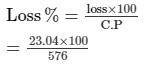= 4%

(ii) S.P of 1 pen = Rs. 2.88

Therefore, S.P of 1 dozen pens = 12x

= 12 × 2.88

= Rs. 34.56

PAGE NO 13.12:

Question 23:

The difference between two selling prices of a shirt at profits of 4% and 5% is Rs 6. Find
(i) C.P. of the shirt
(ii) the two selling prices of the shirt

Let the C.P of both the shirts be Rs.  x .C.P = Rs.  x

For shirt 1:

Profit is 4%:

Profit% = Profit/CP × 100

Profit = 4/100 × C.P

=  Rs. 0.04 x S.P

= C.P + Profit = x + 0.04

x =  Rs. 1.04 x

For shirt 2:Profit  =  5%:

C.P =  Rs.  x

Profit = 5/100 × C.P =  Rs. 0.05 x

S.P = C.P + Profit = x + 0.05

x =  Rs. 1.05 x

It is given that the difference between their profits is Rs. 6

So, 1.05 x −1.04 x = 6

0.01 x = 6

x =  Rs. 600

Thus, C.P = Rs. 600

S.P of shirt 1 = Rs. 1.04 x

= Rs. 1.04 × 600

= Rs. 624

S.P of shirt 2 =  Rs.1.05 x

=  Rs.1.05 × 600

= Rs. 630.

PAGE NO 13.12:

Question 24:

Toshiba bought 100 hens for Rs 8000 and sold 20 of these at a gain of 5%. At what gain percent she must sell the remaining hens so as to gain 20% on the whole?

C.P of 100 hens = Rs. 8000

Cost of one hen = 8000/100 = Rs. 80

C.P of 20 hens  = Rs.( 80 × 20) = Rs. 1600

Gain% = 5%

S.P = C.P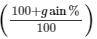S.P = 1600 × 105/100 = Rs. 1680

C.P of 80 hens = Rs.(80 × 80) = Rs.6400

Gain on 80 hens = S.P of 80 hens−C.P of 80 hens

Gain on 100 hens  = Gain on 80 hens + Gain on 20 hens

Gain on 100 hens  = Rs.(80 + S.P of 80 hens − 6400)

Gain% on 100 hens =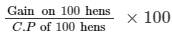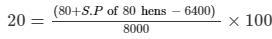1600 = 80 + S.P of 80 hens − 6400

S.P of 80 hens = Rs.( 1600 + 6400−80)S.P of 80 hens = Rs. 7920

Gain on 80 hens =  S.P of 80 hens −C.P of 80 hens = Rs.(7920−6400) = Rs.1520

Gain% on 80 hens  = Gain on 80 hens

C.P of 80 hens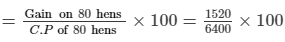= 23.75%

Therefore, Toshiba gained 23.75% on 80 hens.

91 docs

,

,

,

,

,

,

,

,

,

,

,

,

,

,

,

,

,

,

,

,

,

,

,

,

,

,

,

,

,

,

;